# Types of Angles

Yuanxin (Amy) Yang Alcocer, DaQuita Hester
• Author
Yuanxin (Amy) Yang Alcocer

Amy has a master's degree in secondary education and has been teaching math for over 9 years. Amy has worked with students at all levels from those with special needs to those that are gifted.

• Instructor
DaQuita Hester

DaQuita has taught high school mathematics for six years and has a master's degree in secondary mathematics education.

What are the types of angles and angle relationships? Learn about corresponding interior angles, alternate corresponding angles, and angle relationships names. Updated: 12/01/2021

Show

## Geometry Angle Relationships

Angles are a big part of geometry. An angle is defined as the amount of turn between two lines, segments, or rays. Some angles are well known. For example, to turn a corner is usually 90 degrees. The corners of a piece of paper are 90-degree corners as the amount of turn to go from one edge to the next is 90 degrees.

Common types of angles include acute, obtuse, right, straight, and reflex angles. Acute angles are the angles under 90 degrees while obtuse angles are those angles greater than 90 degrees. A 90-degree angle is called a right angle. A 180-degree angle is called a straight angle. And reflex angles are those angles greater than 180 degrees but less than 360 degrees.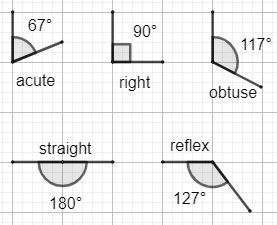Putting two angles together, there are two special circumstances. The first is called complementary angles where the pair of angles form a 90-degree angle together. For example, a 40-degree and 50-degree angle together forms a complimentary pair of angles because they add up to 90 degrees. A supplementary pair of angles is a pair that adds up to 180 degrees. For example, a 110-degree angle and a 70-degree angle form a supplementary pair of angles because they add up to 180 degrees.

In addition to these special situations involving two angles, there are even more angle relationships when two lines intersect a third forming a total of eight angles.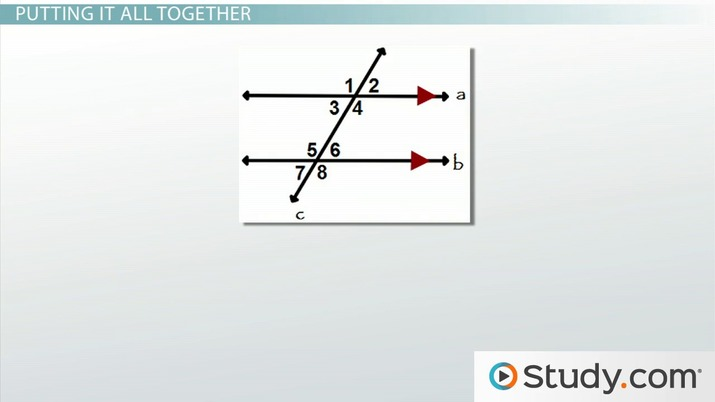An error occurred trying to load this video.

Try refreshing the page, or contact customer support.

Coming up next: Angles and Triangles: Practice Problems

### You're on a roll. Keep up the good work!

Replay
Your next lesson will play in 10 seconds
• 0:05 Introduction
• 0:43 Types of Angles
• 2:30 Determining Angle…
• 4:03 Angle Measures
• 4:53 Putting It All Together
• 9:24 Lesson Summary
Save Save

Want to watch this again later?

Timeline
Autoplay
Autoplay
Speed Speed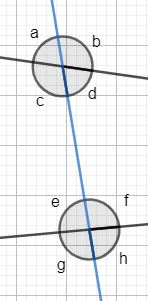## Types of Angle Relationships and Angle Relationship Names

There are six types of angle relationships. Here is a table showing the types of angle relationships and the angle relationships' names. Use the above-angle picture for reference.

Angle Relationship Example
Corresponding Angles Angles a and e
Alternate interior angles Angles d and e
Alternate exterior angles Angles b and g
Consecutive interior angles Angles d and f
Consecutive exterior angles Angles b and h
Vertical angles Angles e and h

The two alternate angles are grouped under alternate angles and the two consecutive angles are grouped under consecutive angles. The interior and exterior designation describes which pair of alternate or consecutive angles are being referred to.

## Alternate and Corresponding Angles

Alternate and corresponding angles are formed when two lines are intersected by a third transversal line. Alternate angles are those angles that are on opposite sides of the transversal (the blue line in the reference picture above). Corresponding angles are those angles that are in the same relative positions at each intersection.

There are two types of alternate angles including alternate interior angles and alternate exterior angles. Both will be discussed in more detail.

### Alternate Interior Angles

Alternate interior angles are the angles between the two lines being intersected and on opposite sides of the transversal. Using the reference picture, there are two pairs of alternate interior angles.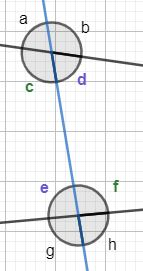The green angles c and f form one pair of alternate interior angles and the purple angles d and e form the second pair of alternate interior angles. Both pairs are inside the two black lines and each angle is on the opposite side of the blue transversal.

### Alternate Exterior Angles

Alternate exterior angles are alternate angles that are outside the two lines being intersected by the transversal. Just like there are two pairs of alternate interior angles, there are also two pairs of alternate exterior angles.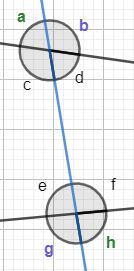One pair of alternate exterior angles are the green angles a and h. Another pair of alternate exterior angles are the purple angles b and g. Both pairs are outside of the two black lines with each angle being on the opposite side of the blue transversal.

### Corresponding Angles: What Do Corresponding Angles Look Like?

Corresponding angles are the angles that are in the same location at each intersection. For example, if one angle is at the NW side of an intersection, then a corresponding angle will also be at the NW side of another intersection. So what do corresponding angles look like?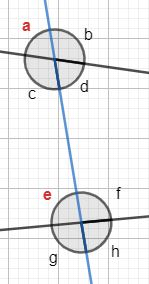The pair of red angles a and e are corresponding angles. Notice how both are in the same location at their respective intersections. Both are at the top left or NW part of the intersection.

An intersection has four sections, so that means there are four pairs of corresponding angles. The other corresponding angles are angles b and f, d and h, and c and g. Notice how they all are located at the same relative positions at their respective intersections.

### Are There Corresponding Interior Angles and Corresponding Exterior Angles?

The question as to whether there are corresponding interior and exterior angles is a common question. This is probably due to the fact that there are alternate interior and exterior angles. But for corresponding angles, this is not the case. Note how there are four corresponding angle pairs where there are only two alternate interior angle pairs and only two alternate exterior angle pairs.

Corresponding angles cannot be all interior nor can they be all exterior. Because they are relative to the location at an intersection, one angle will always be interior while the other angle will always be exterior. If both angles are interior, then they cannot be corresponding since their relative position at an intersection has changed.

## Consecutive Interior Angles

Consecutive interior angles are interior angles that are on the same side of the transversal. These angles are not on opposite sides of the transversal.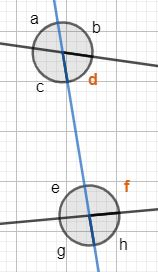The orange angles d and f make up a consecutive interior angle pair. Both are interior angles being inside of the two black lines and both are on the same side of the blue transversal. There is one more pair of consecutive interior angles. The angles c and e make up that other pair. Just like there are two sets of alternate interior angles, there are two sets of consecutive interior angles.

## Consecutive Exterior Angles

Consecutive exterior angles are exterior angles that are on the same side of the transversal.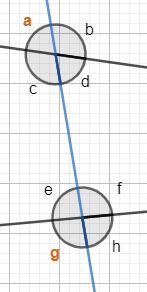The orange angles a and g make up a consecutive exterior angle pair. Just like there are two pairs of consecutive interior angles, there are also two pairs of consecutive exterior angles. The other pair are the angles b and h. Both these angle pairs are outside the two black lines and both pairs are on the same side of the blue transversal.

To unlock this lesson you must be a Study.com Member.

#### How do you find alternate and corresponding angles?

Alternate angles are on opposite sides of a transversal. Alternate interior angles are alternate angles between the two lines intersected by the transversal. Alternate exterior angles are the alternate angles outside the two lines intersected by the transversal.

#### How do you solve for corresponding interior angles?

Because corresponding angles are at the same relative positions at their intersections, there are no corresponding interior or exterior angles. If the transversal intersects two parallel lines, then the corresponding angles will be congruent.

#### Are corresponding interior angles congruent?

Corresponding interior angles are congruent when the transversal intersects two parallel lines. When this happens, it creates two identical intersections.

#### What are the three angle relationships?

There are corresponding angles, alternate angles, and consecutive angles for transversals that intersect two lines. Vertical angles exist for any intersection between two lines.

#### Are corresponding angles supplementary or complementary?

Corresponding angles will be supplementary when the transversal intersects two parallel lines perpendicularly. When this happens, the corresponding angles will each measure 90 degrees. Otherwise, if the transversal intersects two parallel lines, then the corresponding angles will be congruent.

### Register to view this lesson

Are you a student or a teacher?